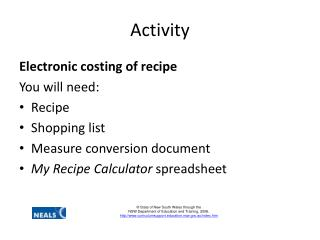DownloadDownload PresentationActivity

# Activity

Download Presentation## Activity

- - - - - - - - - - - - - - - - - - - - - - - - - - - E N D - - - - - - - - - - - - - - - - - - - - - - - - - - -
##### Presentation Transcript

1. Activity Electronic costing of recipe You will need: Recipe Shopping list Measure conversion document My Recipe Calculator spreadsheet

2. Recipe calculator • Open the file recipecalc.xls • Save as: Yourname_recipecalc • To unlock the spreadsheet go to Review>Unprotect sheet. Type in finlit.

3. Type in the ingredients from the recipe

4. Type in the quantities listed in the recipe. Use the Internet to locate measure information for each ingredient to calculate ingredients in grams and millilitres. What is meant by the term net weight? Access an online supermarket website, grocery dockets or visit a supermarket to identify purchase quantity* and cost per purchase unit *The purchase quantity is the package size of the ingredient purchased .e.g. 475 gm can, 1 kilogram (1000 grams) flour, 250 grams butter

5. The spreadsheet will calculate the total cost for each ingredient used in the recipe. The formula is purchase quantity cost divided by purchased quantity divided by the required measure. Click into any cell address in the column Total Cost to see the spreadsheet formula. =E7(D7/C7) *What does the term unit cost mean?

6. Insert number of serves in the pale pink cell. Cost per serve will be calculated.Total cost is divided by the number of serves. Total cost is calculated automatically =F22/F23

7. Discuss a marketable selling price. Insert into pale pink cell. Profit per serve is calculated. Profit per serve= Selling price per serve - Cost per serve Enter selling price per serve =I22-F24

8. Percentage mark up is then calculated. This is calculated by profit per serve over cost per serve as a percentage. E.g. If cost per serve is \$1.00 and the selling price per serve is \$2.00. What is the profit? This cell has been formatted to display as a percentage